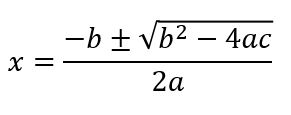# Factoring, Parabolas & Quadratics Flashcards

1 / 33 0 0
33 cards in set

## Flashcard Content Overview

In this flashcard set, you will find definitions to all the terms related to graphing parabolas and factoring quadratics. FOIL is explained with definitions and examples. You will find cards to help you identify the parts of a parabolic graph from the quadratic equation it stems from. You will also find example problems and definitions to cement your understanding of completing the square. When you have mastered these flashcards, you should be ready for your exam on Factoring, Parabolas & Quadratics.

Front
Back
Characteristics of a Parabola:
• Looks like a U shape
• Has 1 vertex
• Always symmetrical
• Shape of the path of a thrown object
Vertex of a Parabola

The point at which a parabola changes direction.

Concave down it is the maximum.

Concave up it is the minimum.

Maximum of a Parabola
Highest point on the U shaped graph.
Type of equation that leads to a parabola graph.
Quadratics with squared terms such as x2.

The graph of which of these equations are parabolas:

a. y = x + 1

b. y = x5 - 1

c. y = x2 - 6

d. y = 2x2

Quadratics with squared terms only lead to parabolas, so c. and d. are parabolas.

Identify the parts of the graph in this vertex form equation.

y= -5(x + 1)2 - 2

-5 = concave down

y-intercept = -7 (substitute x = 0)

axis of symmetry x = - 1 (from equation)

Axis of Symmetry
Line at which a graph could be folded and it would be the same on both sides. It goes straight down the middle.

Identify the parts of the graph in this standard form equation:

y = ax2 + bx + c

y = ax2 + bx + c

a = concave up (pos) or down(neg)

y-intercept = c

axis of symmetry: x = -b/2a

vertex: sub x = -b/2a in to get y value for coordinate point (x,y)

Find the vertex of: y = 3x2 + 6x +1

Axis of sym: x = -(6)/2(3) = -1

Sub: y = 3(-1)2 + 6(-1) + 1 = -2

Vertex point (-1,-2)

FOIL

A binomial (expression with two terms) is multiplied by a binomial.

Multiply: First terms in each binomial, Outside terms, Inside terms, then Last terms.

Multiply:

(x - 5)(x + 2)

F: x * x = x2

O: x*2=2x

I: -5 * x = -5x

L: -5 * 2 = -10

x2 - 3x - 10Inside the sqrt sign: b2 - 4ac
Use the quadratic formula to determine the discriminant of: x2 - 3x +1

Discriminant of Quadratic Formula is b2 - 4ac

-32 - 4(1)(1) = 5

The Standard Form of a Quadratic Equation
y = ax2 + bx + c
Process to solve quadratics that are not in standard form.
Use algebraic rules to rewrite the equation in proper standard form, then solve with the zero product property or the quadratic formula.

Solve:

(x+2)2 = 8 + 4x

Expand and solve for standard form:

x2 + 4x + 4 - 8 - 4x = 0

x2 - 4 = 0

Solve with zero product

(x - 2)(x + 2) = 0

x = 2 or -2

Zero Product Property
Solving quadratics by factoring into a product of binomials equal to zero. This offers two binomials to set equal to zero in order to get solutions to the original quadratic.

Find the solutions:

x2 - 3x + 2 = 0

Factor into binomials:

(x - 2)(x - 1) = 0

x - 2 = 0

x - 1 = 0

x = 2 and x = 1

Root of a Function
A solution when the function equals 0. The roots of a function are the numbers, which when multiplied together give the original function.

Give the roots of this function:

f(x) = x2 - 4x + 4

The roots are both 2.

(x - 2)(x - 2) = 0

x - 2 = 0

x = 2

Factors

Values that when multiplied together give an original number.

Ex: the factors of 15 are 5 and 3 (5*3=15)

Product
Process to factor quadratics, using x2 + 7x + 6 as an example.

Factor constant term (6): 6*1, 2*3, -6*-1, -2*-3

Add factors to find middle term number (7x): 6 + 1 = 7

Separate factors into product of binomials

(x + 6)(x + 1)

Factor: x2 - 4

constant factors: 2 * -2

sum of factors=0=middle term of (0x)

use factors in binomials

(x + 2)(x - 2)

Purpose of completing the square
To change a standard form equation into a vertex form equation.
Perfect Square Binomial
A binomial that is squared: (x - h)2.
A trinomial can be factored into a Perfect Square when...

ax2 + bx + c

and

(b/2)2 = c

Process of completing the square
Change the c (using algebraic rules) so that it equals (b/2)2.

Solve using complete the square:

x2 + 2 = 6x - 2

1. Standard form: x2 - 6x + 4 = 0

2. (b/2)2 = (-6/2)2 = 9

3. to make c = 9 add 5 to both sides

4. x2 - 6x + 9 = 5

5. factor perfect square and solve (x - 3)(x - 3) = 5

x - 3 = 5

x = 8

The coefficient (number being multiplied) of the first term in a polynomial.
The process of factoring non-1 leading coeffecient trinomials.

Need 2 numbers whose sum is the middle coefficient.

Product of the numbers =product of the constant and the leading coefficient.

Work backwards to get correct coefficients for each binomial.

Factor: 3x2 - 14x - 5

_+_ = -14

_*_ = (3 * -5) = -15

numbers are 1 and -15

factor -15 (3 * -5)

final: (3x + 1)(x - 5)

To unlock this flashcard set you must be a Study.com Member.